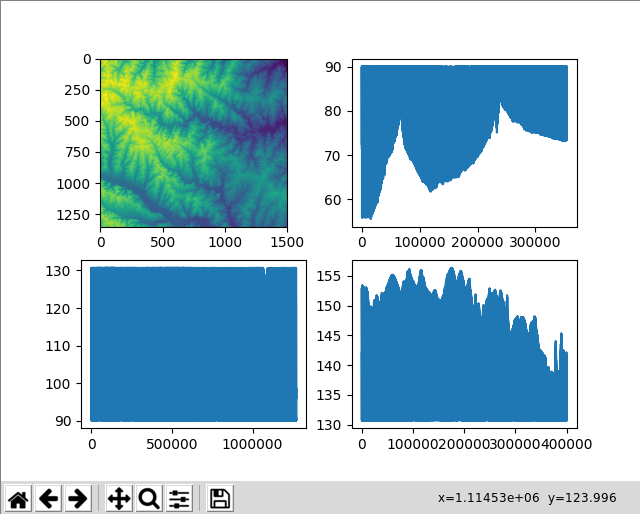# How to extract cell values from a GeoTIFF file using NumPy

Institute for Environmental and Spatial Analysis...University of North Georgia

## 1   Introduction

Try these exercises first:

In this exercise, we want to extract cell values that satisfy certain conditions. Our goal is not to create subsets of a GeoTIFF file as 2-dimensional maps. Instead, we’re only interested in their cell values, not their locations. Use elevation.tif. To extract cells while retaining their location information, see this exercise.## 2   Python code

``````from osgeo import gdal
import matplotlib.pyplot as plt

ds = gdal.Open(filename)
band = ds.GetRasterBand(1)
del ds, band
return arr

elev_mean = elev_arr.mean()
elev_std = elev_arr.std()

print("# Elevation statistics")
print("The mean elevation is", elev_mean)
print("The standard deviation of elevation is", elev_std)

low_band = elev_arr[elev_arr < elev_mean-elev_std]
middle_band = elev_arr[(elev_arr >= elev_mean-elev_std) &
(elev_arr <= elev_mean+elev_std)]
high_band = elev_arr[elev_arr > elev_mean+elev_std]

print()
print("# Low band statistics")
print("The mean elevation of the low band is", low_band.mean())
print("The xtandard deviation of the low band is", low_band.std())

print()
print("# Middle band statistics")
print("The mean elevation of the middle band is", middle_band.mean())
print("The xtandard deviation of the middle band is", middle_band.std())

print()
print("# High band statistics")
print("The mean elevation of the high band is", high_band.mean())
print("The xtandard deviation of the high band is", high_band.std())

# upper left
plt.subplot(221)
plt.imshow(elev_arr)

# upper right
plt.subplot(222)
plt.plot(low_band)

# bottom left
plt.subplot(223)
plt.plot(middle_band)

# bottom right
plt.subplot(224)
plt.plot(high_band)

plt.show()``````

## 3   Explanation

We need these two modules.

``````from osgeo import gdal
import matplotlib.pyplot as plt``````

Define the `read_geotiff()` function that reads a GeoTIFF and its NumPy array.

``````def read_geotiff(filename):
ds = gdal.Open(filename)
band = ds.GetRasterBand(1)
del ds, band
return arr``````

Now, call this function to read a GeoTIFF file and get a NumPy array.

``elev_arr = read_geotiff("elevation.tif")``

Using the `mean()` and `std()` functions in the NumPy array class, calculate the mean and standard deviation of the elevation data.

``````elev_mean = elev_arr.mean()
elev_std = elev_arr.std()``````

Print the elevation statistics. The `print()` function can take an arbitrary number of arguments to print. For example, `print("The mean elevation is", elev_mean)` prints the string `The mean elevation is` and a number in `elev_mean` separated by a space.

``````print("# Elevation statistics")
print("The mean elevation is", elev_mean)
print("The standard deviation of elevation is", elev_std)``````

Extract elevation cell values that are less than the mean minus standard deviation. Here, we use `elev_arr < elev_mean-elev_std` to create a boolean array for indexing `elev_arr`. The shape of this boolean array is the same as that of `elev_arr`. `elev_arr[elev_arr < elev_mean-elev_std]` returns `elev_arr` cell values whose boolean counterpart in `elev_arr < elev_mean-elev_std` is `True`. It returns a 1-dimensional array.

``low_band = elev_arr[elev_arr < elev_mean-elev_std]``

For the middle band, we need to extract cell values that are between two numbers: the mean minus standard deviation and the mean plus standard deviation. In this case, we cannot use the regular `and` combine `>=` and `<=` conditions because this operator takes two `bool` operands, but conditioning boolean arrays are not of the `bool` type, but they are arrays of `bool`. The `&` operator combines two boolean arrays element-wise and create a new boolean array of the same shape. In this case, the shapes of `elev_arr >= elev_mean-elev_std` and `elev_arr <= elev_mean+elev_std` are the same as that of `elev_arr`. We use parentheses `()` to make sure that each boolean operand is evaluated first. Without parentheses, the `&` operator will take `elev_mean-elev_std` and `elev_arr`, which have different shapes.

``````middle_band = elev_arr[(elev_arr >= elev_mean-elev_std) &
(elev_arr <= elev_mean+elev_std)]``````

Last, we extract high-band elevation cells.

``high_band = elev_arr[elev_arr > elev_mean+elev_std]``

`print()` prints an empty line to separate output lines.

``````print()
print("# Low band statistics")
print("The mean elevation of the low band is", low_band.mean())
print("The xtandard deviation of the low band is", low_band.std())

print()
print("# Middle band statistics")
print("The mean elevation of the middle band is", middle_band.mean())
print("The xtandard deviation of the middle band is", middle_band.std())

print()
print("# High band statistics")
print("The mean elevation of the high band is", high_band.mean())
print("The xtandard deviation of the high band is", high_band.std())``````

Let’s plot these data in a 2-by-2 grid. `plt.subplot(221)` means that we want to create a 2-by-2 grid (the first `22`) and place the following command at index 1. Subfigure indexing starts from the upper-left corner using 1-based indexing and grows towards the right and then the bottom.

``````# upper left
plt.subplot(221)
plt.imshow(elev_arr)

# upper right
plt.subplot(222)
plt.plot(low_band)

# bottom left
plt.subplot(223)
plt.plot(middle_band)

# bottom right
plt.subplot(224)
plt.plot(high_band)

plt.show()``````